The Sundial Primer created by Carl SabanskiThe Sundial Primer Index "Sunny Day U" Index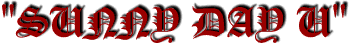Make a Horizontal Sundial - Graphical Method 3

Horizontal Sundial: the common or garden sundial with a horizontal dial plate and polar-pointing gnomon.

Here is yet another graphical method that you can use to draw a horizontal sundial. Using this method it easier to obtain hour lines that are earlier than 7 a.m. and later than 5 p.m.. A number of steps are similar to the first graphical method presented and are combined.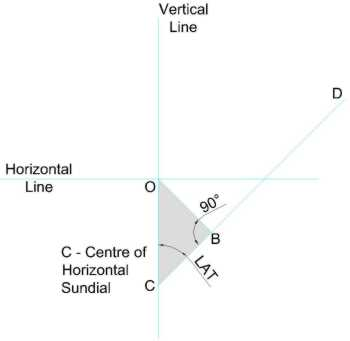Step 1

• Draw a horizontal line.

• Draw a vertical line that passes through the horizontal line at O.

• Select a point C on the vertical line. This point will be the centre of your horizontal sundial and eventually all the hour lines will radiate from it.

• Draw the line CD at an angle equal to the latitude (LAT) from OC.

• Draw the line OB from O and perpendicular to line CD. The line OB is the radius of the equatorial sundial you will be drawing next.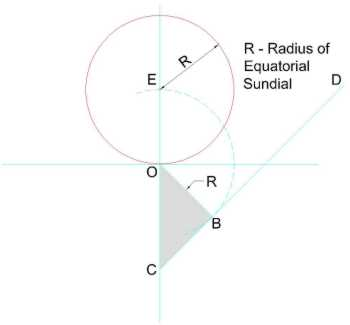Step 2

• Place your compass point at O and draw an arc from B until it crosses the vertical line at E. This is the centre of your equatorial sundial.

• Place your compass point at E and draw a circle starting at O. Notice that OE=OB=R. You have now drawn the face of your equatorial sundial.Step 3

• Draw the equatorial sundial hour lines spaced at 15° in both directions but do not go beyond 9 a.m. and 3 p.m..

• If you want smaller time intervals add more lines. Remember that 15°=60 minutes so 7.5°=30 minutes, 1°=4 minutes. You can figure out the rest.

• Extend all the lines to the horizontal line.

• From C, the centre of the horizontal sundial, draw a line to each of the points on the horizontal line. You have now transferred the hour lines from the equatorial sundial to the horizontal sundial.

• Draw the line JK from the 9 a.m. point and LM from the 3 p.m. point. These lines are parallel to line OC.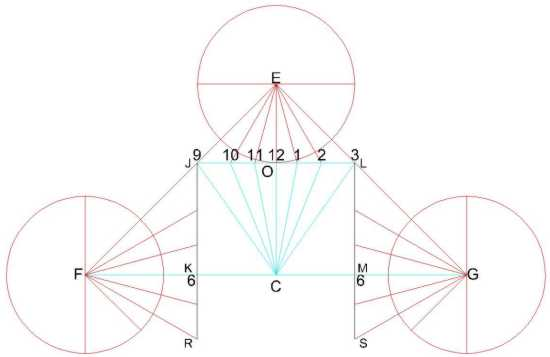Step 4

• Extend the horizontal lines CK and CM to points F and G where the distances CF and CG are equal to the distance CE.

• Draw a circle with centre F and radius OE.

• Draw lines on either side of FK spaced at 15° intervals. If you want smaller time intervals add more lines.

• Extend these lines to the line JK.

• Extend the line JK to the point R so all the hour points are on the line JKR.

• Draw a circle with centre G and radius OE.

• Draw lines on either side of GM spaced at 15° intervals. If you want smaller time intervals add more lines.

• Extend these lines to the line LM.

• Extend the line LM to the point S so all the hour points are on the line LMS.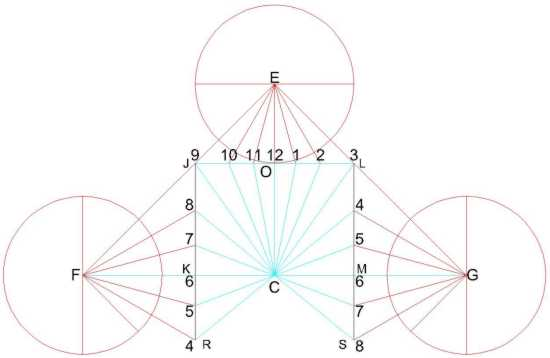Step 5

• From C, the centre of the horizontal sundial, draw a line to each of the points on lines JKR and LMS. You have now transferred the remaining hour lines to the horizontal sundial.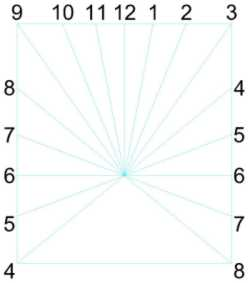Step 6

• The remaining steps are the same as described in the first graphical method presented.

This is a very interesting method of drawing a horizontal sundial and it is very easy to draw, for example, the 6:15 a.m. hour line.

Happy Dialling!